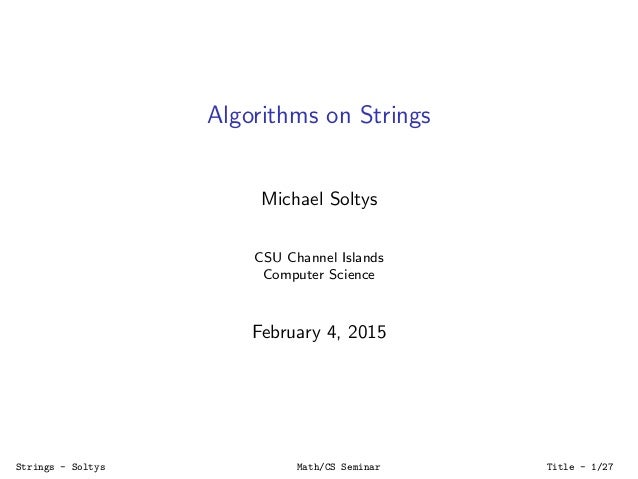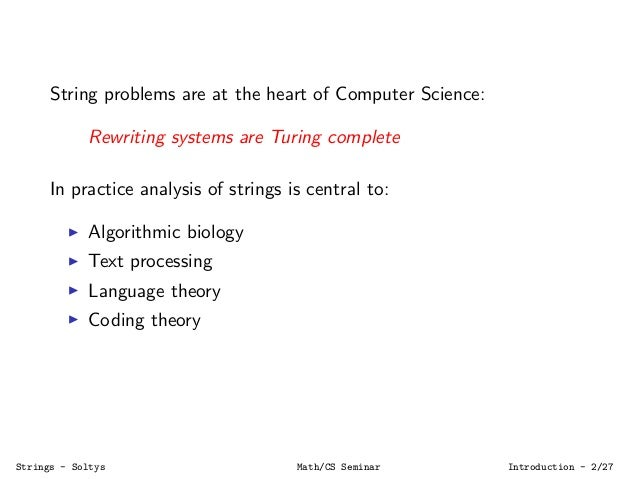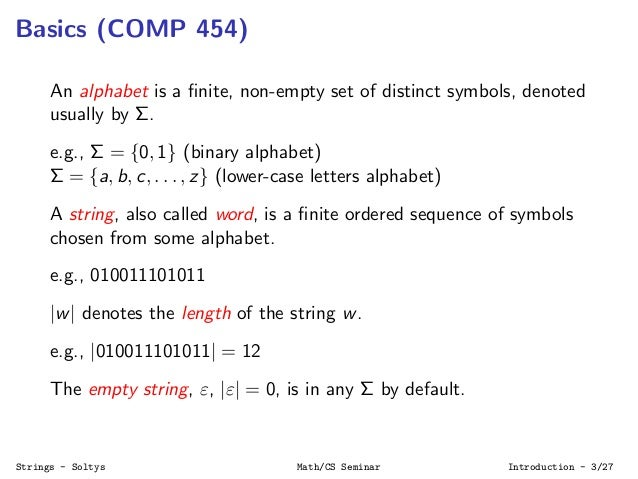Successfully reported this slideshow.Upcoming SlideShare
×

of0

Share

# Algorithms on Strings

This talk is going to be centered on two papers that are going to appear in the following months:

Neerja Mhaskar and Michael Soltys, Non-repetitive strings over alphabet lists
to appear in WALCOM, February 2015.

Neerja Mhaskar and Michael Soltys, String Shuffle: Circuits and Graphs
to appear in the Journal of Discrete Algorithms, January 2015.

Visit http://soltys.cs.csuci.edu for more details (these two papers are number 3 and 19 on the page), as well as Python programs that can be used to illustrate the ideas in the papers. We are going to introduce some basic concepts related to computations on string, present some recent results, and propose two open problems.

See all

### Related Audiobooks

#### Free with a 30 day trial from Scribd

See all
• Be the first to like this

### Algorithms on Strings

1. 1. Algorithms on Strings Michael Soltys CSU Channel Islands Computer Science February 4, 2015 Strings - Soltys Math/CS Seminar Title - 1/27
2. 2. String problems are at the heart of Computer Science: Rewriting systems are Turing complete In practice analysis of strings is central to: Algorithmic biology Text processing Language theory Coding theory Strings - Soltys Math/CS Seminar Introduction - 2/27
3. 3. Basics (COMP 454) An alphabet is a ﬁnite, non-empty set of distinct symbols, denoted usually by Σ. e.g., Σ = {0, 1} (binary alphabet) Σ = {a, b, c, . . . , z} (lower-case letters alphabet) A string, also called word, is a ﬁnite ordered sequence of symbols chosen from some alphabet. e.g., 010011101011 |w| denotes the length of the string w. e.g., |010011101011| = 12 The empty string, ε, |ε| = 0, is in any Σ by default. Strings - Soltys Math/CS Seminar Introduction - 3/27
4. 4. Σk is the set of strings over Σ of length exactly k. e.g., If Σ = {0, 1}, then Σ0 = {ε} Σ1 = Σ Σ2 = {00, 01, 10, 11}, etc. |Σk |? Kleene’s star Σ∗ is the set of all strings over Σ. Σ∗ = Σ0 ∪ Σ1 ∪ Σ2 ∪ Σ3 ∪ . . . =Σ+ Concatenation If x, y are strings, and x = a1a2 . . . am & y = b1b2 . . . bn ⇒ x · y = xy juxtaposition = a1a2 . . . amb1b2 . . . bn UNIX cat command Strings - Soltys Math/CS Seminar Introduction - 4/27
5. 5. A language L is a collection of strings over some alphabet Σ, i.e., L ⊆ Σ∗. E.g., L = {ε, 01, 0011, 000111, . . .} = {0n 1n |n ≥ 0} (1) Note: wε = εw = w. {ε} = ∅; one is the language consisting of the single string ε, and the other is the empty language. Strings - Soltys Math/CS Seminar Introduction - 5/27
6. 6. Consider L = {w| w is of the form x01y ∈ Σ∗ } where Σ = {0, 1}. We want to specify a DFA A = (Q, Σ, δ, q0, F) that accepts all and only the strings in L. Σ = {0, 1}, Q = {q0, q1, q2}, and F = {q1}. Transition diagram q 1 0 0,1 10 q0 q2 1 Transition table 0 1 q0 q2 q0 q1 q1 q1 q2 q2 q1 Strings - Soltys Math/CS Seminar Introduction - 6/27
7. 7. A context-free grammar (CFG) is G = (V , T, P, S) — Variables, Terminals, Productions, Start variable Ex. P −→ ε|0|1|0P0|1P1. Ex. G = ({E, I}, T, P, E) where T = {+, ∗, (, ), a, b, 0, 1} and P is the following set of productions: E −→ I|E + E|E ∗ E|(E) I −→ a|b|Ia|Ib|I0|I1 If αAβ ∈ (V ∪ T)∗, A ∈ V , and A −→ γ is a production, then αAβ ⇒ αγβ. We use ∗ ⇒ to denote 0 or more steps. L(G) = {w ∈ T∗|S ∗ ⇒ w} Strings - Soltys Math/CS Seminar Introduction - 7/27
8. 8. Context-sensitive grammars (CSG) have rules of the form: α → β where α, β ∈ (T ∪ V )∗ and |α| ≤ |β|. A language is context sensitive if it has a CSG. Fact: It turns out that CSL = NTIME(n) A rewriting system (also called a Semi-Thue system) is a grammar where there are no restrictions; α → β for arbitrary α, β ∈ (V ∪ T)∗. Fact: It turns out that a rewriting system corresponds to the most general model of computation; i.e., a language has a rewriting system iﬀ it is “computable.” Strings - Soltys Math/CS Seminar Introduction - 8/27
9. 9. A second course in Automata Chomsky-Schutzenberger Theorem: If L is a CFL, then there exists a regular language R, an n, and a homomorphism h, such that L = h(PARENn ∩ R). Parikh’s Theorem: If Σ = {a1, a2, . . . , an}, the signature of a string x ∈ Σ∗ is (#a1(x), #a2(x), . . . , #an(x)), i.e., the number of ocurrences of each symbol, in a ﬁxed order. The signature of a language is deﬁned by extension; regular and CFLs have the same signatures. Strings - Soltys Math/CS Seminar Introduction - 9/27
10. 10. This presentation is about algorithms on strings. Based on two papers that are coming out in the next months: Neerja Mhaskar and Michael Soltys Non-repetitive strings over alphabet lists to appear in WALCOM, February 2015. Neerja Mhaskar and Michael Soltys String Shuﬄe: Circuits and Graphs accepted in the Journal of Discrete Algorithms, 2015 Both at http://soltys.cs.csuci.edu (papers 3 & 19) Strings - Soltys Math/CS Seminar Introduction - 10/27
11. 11. Non-repetitive strings A word is non-repetitive if it does not contain a subword of the form vv. Word with repetition 010101110 Word without repetition 101 Easy observation: what is the smallest n so that any word over Σ = {0, 1} of length ≥ n has at least one repetition? Strings - Soltys Math/CS Seminar Non-repetitive strings - 11/27
12. 12. Original Thue problem For Σ3 = {1, 2, 3} and morphism, due to A. Thue: S =    1 → 12312 2 → 131232 3 → 1323132 Given a string w ∈ Σ∗ 3, we let S(w) denote w with every symbol replaced by its corresponding substitution: S(w) = S(w1w2 . . . wn) = S(w1)S(w2) . . . S(wn) Lemma: If w is non-repetitive then so is S(w). Strings - Soltys Math/CS Seminar Non-repetitive strings - 12/27
13. 13. Problem extended to alphabet lists List of alphabets L = L1, L2, . . . , Ln Can we generate non-repetitive words w = w1w2 . . . wn, such that the symbol wi ∈ Li ? Studied by: [GKM10], [Sha09], and it is a natural extension of the original problem posed and solved by A. Thue. E.g., L1 = {a, b, c}, L2 = {b, c, d}, L3 = {a, d, 2}, in this case w = ac2 is over L1, L2, L3 and non-repetitive. Is that true for any list where |Li | = 3 for all i? Strings - Soltys Math/CS Seminar Non-repetitive strings - 13/27
14. 14. [GKM10] shows that this can be done for |Li | = 4 for all i with this algorithm: pick any w1 ∈ L1 for i + 1 (w = w1w2 . . . wi is non-repetitive) pick a ∈ Li+1 if wa is non-repetitive, then let wi+1 = a if wa has a square vv, then vv must be a suﬃx delete the right copy of v from w, and restart. Using sophisticated Lov´asz Local Lemma argument and Catalan numbers we can show that the above algorithm succeeds with non-zero probability. Strings - Soltys Math/CS Seminar Non-repetitive strings - 14/27
15. 15. Particular “yes” cases for L1, L2, . . . , Ln Has a system of distinct representatives (SDR) Has the union property Can be mapped consistently to Σ3 = {1, 2, 3} It is a partition Strings - Soltys Math/CS Seminar Non-repetitive strings - 15/27
16. 16. Open Problem 1 Given any list L1, L2, . . . , Ln, where |Li | = 3, can we always ﬁnd a non-repetitive string w over such a list? Strings - Soltys Math/CS Seminar Non-repetitive strings - 16/27
17. 17. Shuﬄe w is the shuﬄe of u, v: w = u v w = 0110110011101000 u = 01101110 v = 10101000 w = 0110110011101000 Strings - Soltys Math/CS Seminar Shuffle - 17/27
18. 18. Shuﬄe w is the shuﬄe of u, v: w = u v w = 0110110011101000 u = 01101110 v = 10101000 w = 0110110011101000 w is a shuﬄe of u and v provided: u = x1x2 · · · xk v = y1y2 · · · yk and w obtained by “interleaving” w = x1y1x2y2 · · · xkyk. Strings - Soltys Math/CS Seminar Shuffle - 17/27
19. 19. Square Shuﬄe w is a square provided it is equal to a shuﬄe of a u with itself, i.e., ∃u s.t. w = u u The string w = 0110110011101000 is a square: w = 0110110011101000 and u = 01101100 = 01101100 Strings - Soltys Math/CS Seminar Shuffle - 18/27
20. 20. Result from 2013 given an alphabet Σ, |Σ| ≥ 7, Square = {w : ∃u(w = u u)} is NP-complete. Strings - Soltys Math/CS Seminar Shuffle - 19/27
21. 21. Result from 2013 given an alphabet Σ, |Σ| ≥ 7, Square = {w : ∃u(w = u u)} is NP-complete. What we leave open: What about |Σ| = 2 (for |Σ| = 1, Square is just the set of even length strings) What about if |Σ| = ∞ but each symbol cannot occur more often than, say, 6 times (if each symbol occurs at most 4 times, Square can be reduced to 2-Sat – see P. Austrin Stack Exchange post http://bit.ly/WATco3) Strings - Soltys Math/CS Seminar Shuffle - 19/27
22. 22. Open Problem 2 Is Square NP-complete for alphabets of size {2, 3, 4, 5, 6} ? Strings - Soltys Math/CS Seminar Shuffle - 20/27
23. 23. Upper and lower bounds Shuﬄe(x, y, w) holds if and only if w is a shuﬄe of x, y Shuﬄe ∈ AC0 , but Shuﬄe ∈ AC1 . Strings - Soltys Math/CS Seminar Shuffle - 21/27
24. 24. Upper bound Strings - Soltys Math/CS Seminar Shuffle - 22/27
25. 25. Lower bound Parity(x) = 0 ≤ i ≤ |x| i is odd Shuﬄe(0|x|−i , 1i , x). Strings - Soltys Math/CS Seminar Shuffle - 23/27
26. 26. n−i i=1 i=3 i=5 i=n 0 x 1 1 10 0 0x x x1 ii n−i i in−i n−i Strings - Soltys Math/CS Seminar Shuffle - 24/27
27. 27. Open Problem 3 Is Shuﬄe in NC1 ? Strings - Soltys Math/CS Seminar Shuffle - 25/27
28. 28. Announcement of two upcoming seminars 1. February 16, 2015, 6:00-7:00pm Bell Tower 1471 Ryszard Janicki On Pairwise Comparisons Based Rankings 2. February 16, 2015, 7:00-8:00pm Bell Tower 1471 Neerja Mhaskar Repetition in Strings and String Shuﬄes Computer Science Seminars: http://compsci.csuci.edu/degrees/seminars.htm Strings - Soltys Math/CS Seminar Conclusion - 26/27
29. 29. References Jaroslaw Grytczuk, Jakub Kozik, and Pitor Micek. A new approach to nonrepetitive sequences. arXiv:1103.3809, December 2010. Jeﬀrey Shallit. A second course in formal languages and automata theory. Cambridge Univeristy Press, 2009. Strings - Soltys Math/CS Seminar References - 27/27

Total views

1,574

On Slideshare

0

From embeds

0

Number of embeds

600

8

Shares

0